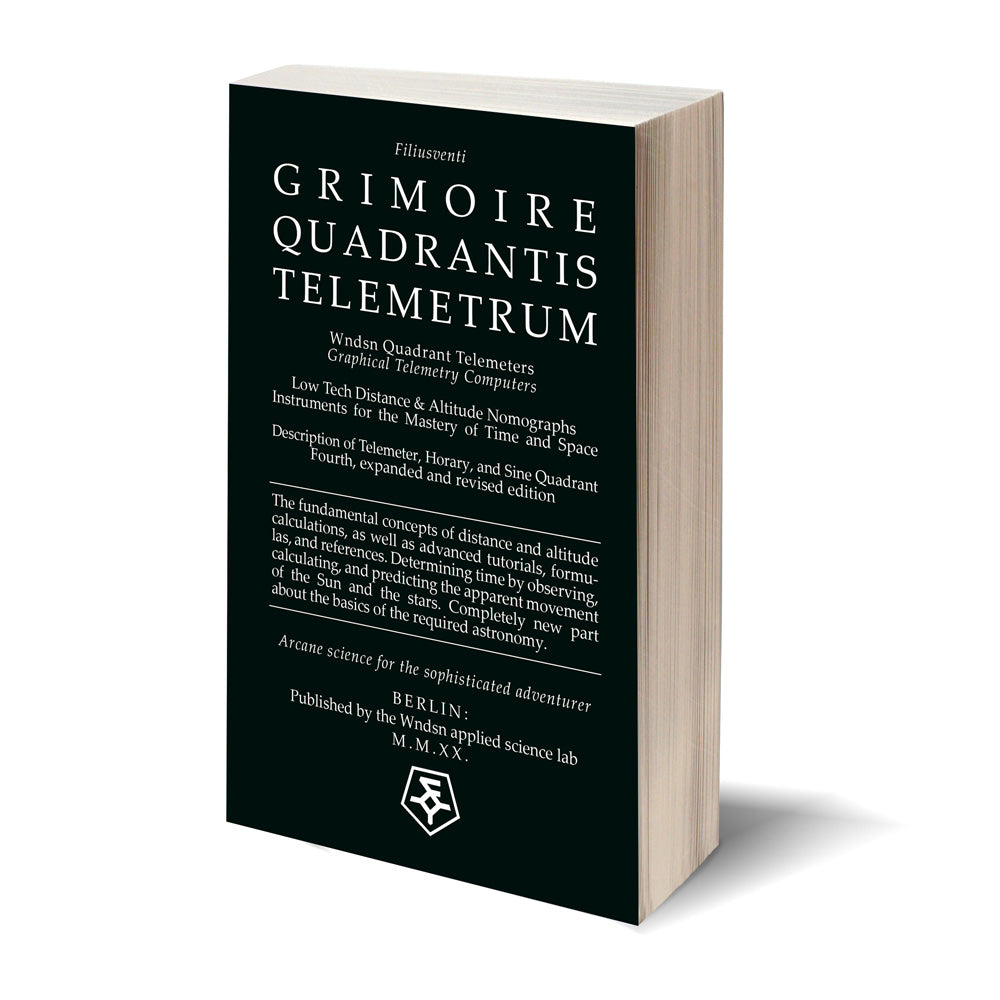# Wndsn Quadrant Telemeters: Official Time & Space Manual (GQT4)

Regular price €24.95 Sale

International shipping available (see our shipping rates and crisis info).

Description and use of the Telemeter, Horary, and Sine Quadrant. Fourth, once again expanded and revised edition.

[Note: If the book is out of stock here, it's available on Amazon.com, Amazon.co.uk, Amazon.de, as well as at your book dealer of choice under the ISBN: 979-8691884504.]

The fundamental concepts of distance and altitude calculations, as well as advanced tutorials, formulas, and reference tables.

The Wndsn Quadrant Telemeter is a simple observational tool for measuring angles via various inputs combined with a number of means to directly process the acquired values. This instrument is equally useful in altimetry, surveying, etc., for taking altitudes, and determining right-angled triangles. This manual is about the theory and practice of the instrument.

This newest edition expands the modern user’s understanding of astronomical systems, providing concise and tangible instruction. The 4th edition reiterates the constituent concepts of calculating distance with reworked fundamental and clarifying graphics; all previous material is included and updated, including the Time & Space addendum that previously introduced setup, history, as well as the functions and tutorials regarding understanding the ancient fundamentals behind the measurement of time and date.

Efficient learning and accessible information is the overarching goal for the 4th edition. The examples and tutorials utilize only the most salient formulas and only require the Quadrant for measurements and computations (plus pen and paper for note-taking). The expanded reference section is a standalone resource for emergency or austere situations. The novice, the seasoned practitioner, and the educator looking to demonstrate mathematical concepts in tangible ways are equally addressed.

Using a Wndsn Quadrant Telemeter to determine latitude (at night with Polaris), calculate declination of the day with the respective arc, and a measurement of the Sun altitude (backsighting), one can derive at least 18 data points and actually calculate all of them directly on the quadrant.

1. Wndsn Quadrant Telemeters - Graphical Telemetry Computers: Official Manual

The manual provides background science and history, set up instructions, reference materials, and advanced tutorials on the following topics:

• Measuring the Depth of a Well
• Finding Tangent
• Measuring from an Elevation
• Measuring an Elevated Object
• Finding Sine and Cosine
• Using the Obliquity Arc
• Extending the Scales
• Powers of 10 and Scale Jumps
• Measuring Angular Size
• Measuring Size Instead of Distance
• Resection: Triangulation, Trilateration, Triangulateration
• Resection Error Margins
• Small-Angle Calculations
• Accuracy Estimations
• Calibrating Device Resolution

Expanded part on astronomy:

• Sun Sighting
• Sun Calculations
• Measuring Local Latitude
• Measuring Sun Altitude
• Using The Obliquity Arc
• Calculating Sun Declination
• Calculating Sun Altitude

• Using the Calendar
• Measuring Time
• Seasonal (“Unequal”) Hours
• Equinoctial (“Equal”) Hours
• Solar Time

• Great Circle Calculations
• Determining Sunrise Bearing
• The Hour Angle
• Sun Azimuth from Hour Angle
• Sun Azimuth from Sun Altitude
• Latitude from Sun Altitude

The update in numbers:

• 140 pages more than the 3rd edition and 30 new topics
• 129 graphics, increase of 57 from the 3rd edition
• 22 tables, increase of 5 from the 3rd edition
• 40 equations, increase of 16 from the 3rd edition

Specifications:

• 398 pages, black and white
• Perfect bound
• 8" x 5" (12.7 x 20.32 cm)